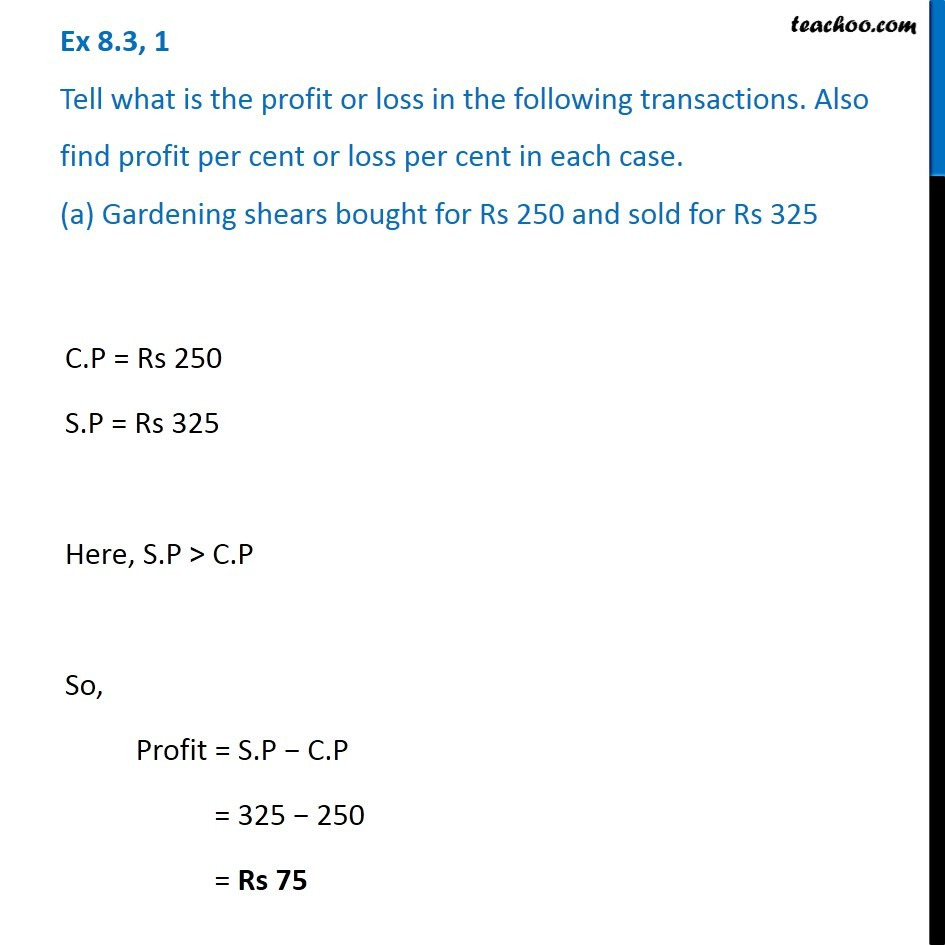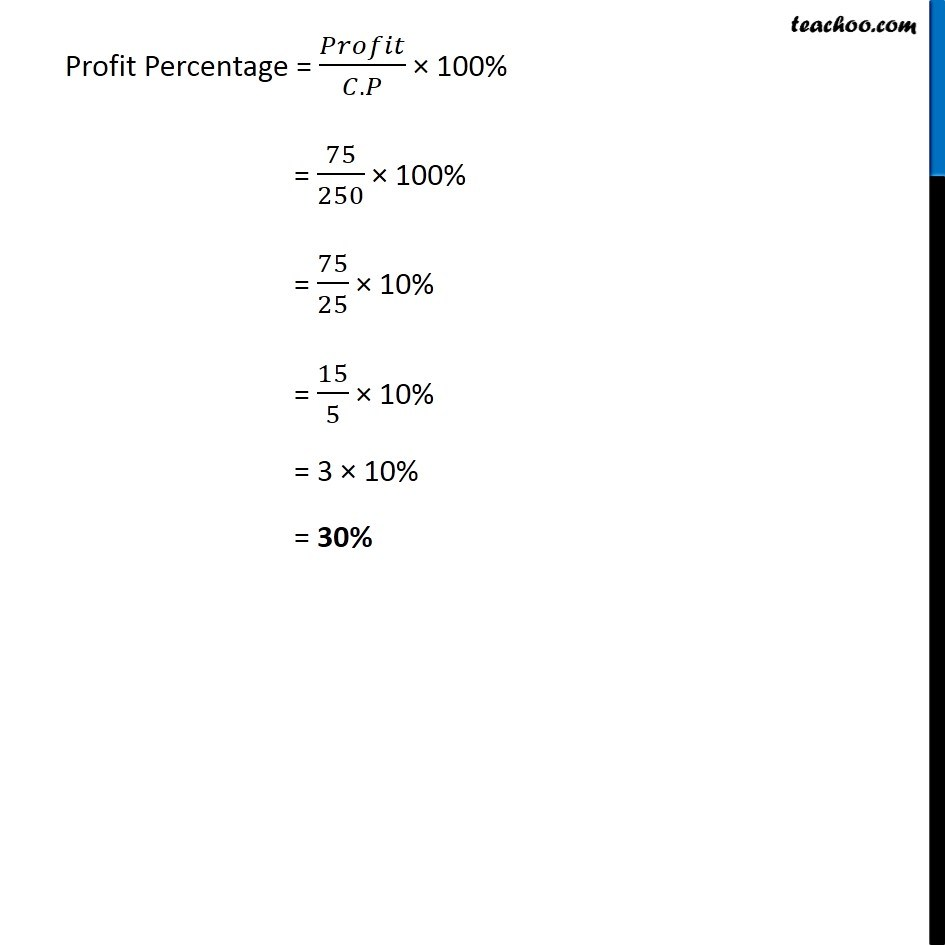Cost price, selling price, profit % , loss%

Chapter 7 Class 7 Comparing Quantities
Concept wiseLearn in your speed, with individual attention - Teachoo Maths 1-on-1 Class

### Transcript

Ex 7.2, 1 Tell what is the profit or loss in the following transactions. Also find profit per cent or loss per cent in each case. (a) Gardening shears bought for Rs 250 and sold for Rs 325 C.P = Rs 250 S.P = Rs 325 Here, S.P > C.P So, Profit = S.P − C.P = 325 − 250 = Rs 75 Profit Percentage = 𝑃𝑟𝑜𝑓𝑖𝑡/(𝐶.𝑃) × 100% = 75/250 × 100% = 75/25 × 10% = 15/5 × 10% = 3 × 10% = 30%